# Digital to Analog Converter or DAC

Op amp is extensively used as main building block of digital to analog convertor. Digital to analog convertor is an electronics device in form of IC, which converts digital signal to its equivalent analog signal. The DAC can be realized in many ways. One of the popular digital to analog convertor circuit is binary weighted ladder.
This is basically a summing amplifier designed with suitable resistances, as shown below.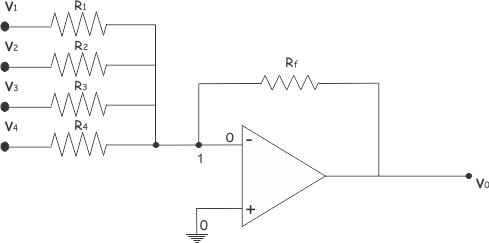Now, applying Kirchhoff Current Law at node 1 of the above circuit, we get,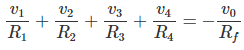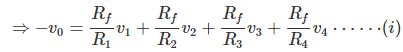Before going through the above circuit of digital to analog convertor, Let us put some suitable values of different resistors connected in the circuit.
Such as, Rf = 10KΩ, R1 = 10KΩ, R2 = 20KΩ, R3 = 40KΩ and R4= 80KΩ.

Putting these values in equation (i) we get,Now, let us also apply voltage at input terminals either 0 or 1 volt. Putting , 0 volt at all inputs,(i.e. v1 = 0, v2 = 0, v3 = 0 and v4 = 0) we get,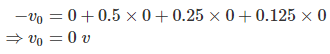So, for digital input 0000, we get analog output 0 volt. Putting, 1V at last input only, (i.e. v1 = 0, v2 = 0, v3 = 0 and v4 = 1V), we get,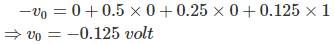Similarly, for v1 = 0, v2 = 0, v3 = 1, v4 = 0For, v1 = 0, v2 = 0, v3 = 1, v4 = 1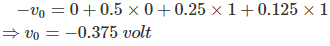In this way the inputs and corresponding outputs can be represented in a table as shown below.

 Binary Input [v1 v2 v3 v4] Decimal Value Output(-v0) 0000 0 0 0001 1 0.125 0010 2 0.25 0011 3 0.375 0100 4 0.5 0101 5 0.625 0110 6 0.75 0111 7 0.875 1000 8 1.0 1001 9 1.125 1010 10 1.25 1011 11 1.375 1100 12 1.5 1101 13 1.625 1110 14 1.75 1111 15 1.875

So, for each decimal number there is one unique output voltage level. From the table it is also seen that, form 0 to 15, for each increment there is an increase of output voltage level by 0.125 volt.
So, the output is analog and it is linearly proportional the decimal equivalent of digital inputs. The above example was of a four bit DAC. A four bit DAC can be represented as shown below.Want To Learn Faster? 🎓
Get electrical articles delivered to your inbox every week.
No credit card required—it’s 100% free.